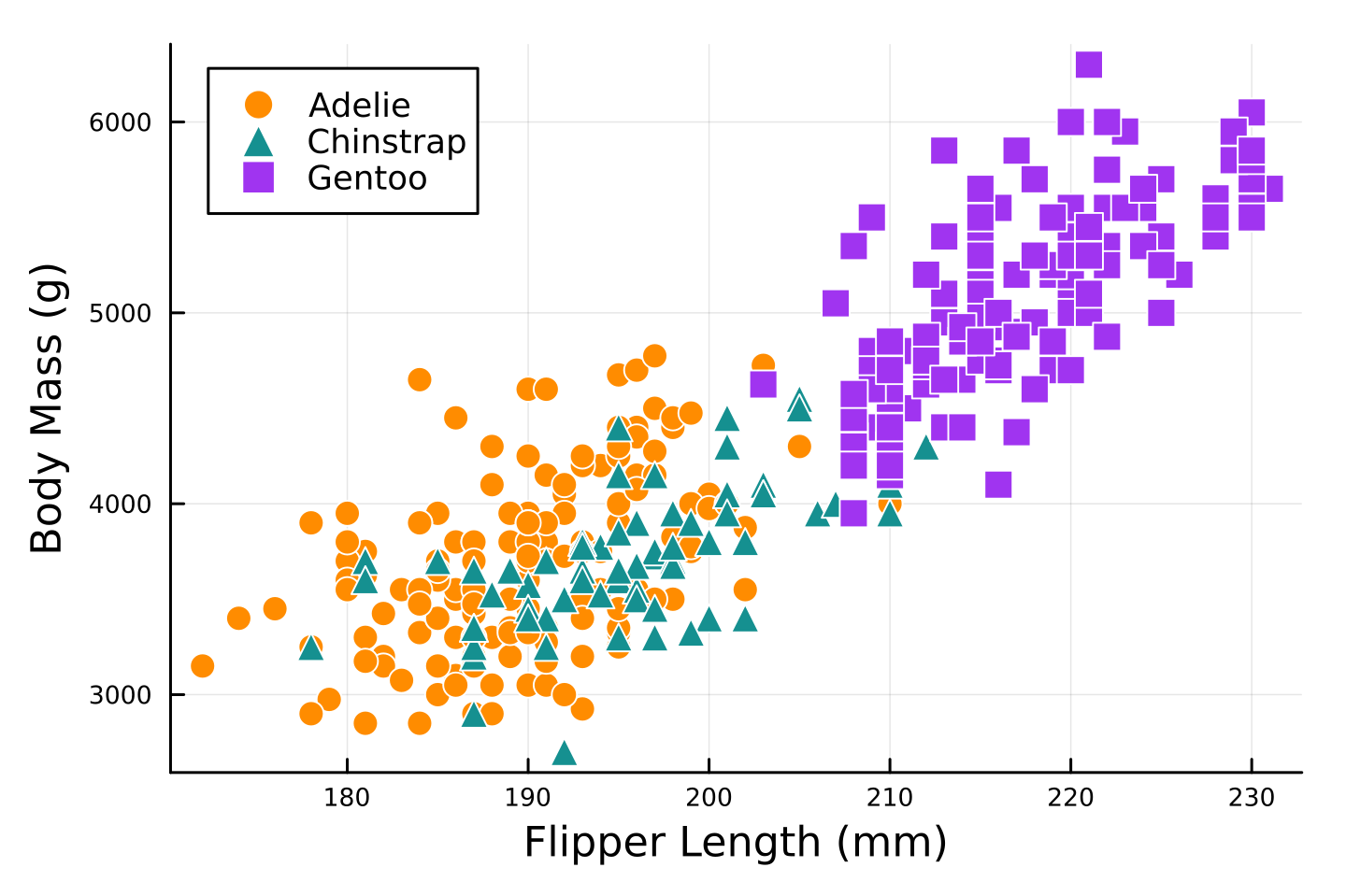# Quarto Is Multi-lingual!

Julia
Python
R
Quarto
Stata
Author

Kazuharu Yanagimoto

Published

April 30, 2023

A common misunderstanding about Quarto is that we cannot use multiple languages within a document. Indeed, Jupyter cannot use multiple languages within a document, and we usually use the jupyter engine for Python and Julia (and it is officially supported.)

However, knitr has already been able to execute multiple languages tons of years ago, so why can’t we do it in Quarto?

Let’s see the following example with `palmerpenguins::penguins` of Horst, Hill, and Gorman (2020)

### R

``````library(palmerpenguins)
library(tidyverse)

ggplot(data = penguins, aes(x = flipper_length_mm)) +
geom_histogram(aes(fill = species),
alpha = 0.5,
position = "identity") +
scale_fill_manual(values = c("darkorange","purple","cyan4")) +
labs(x = "Flipper length (mm)",
y = "Frequency",
title = "Penguin Flipper Lengths",
fill = NULL) +
theme_minimal() +
theme(panel.grid.major.x = element_blank(),
panel.grid.minor = element_blank(),
legend.position = c(0.9, 0.9))``````

### Python

To run a python code on knitr, `install.packages("reticulate")`

``````from palmerpenguins import load_penguins
import seaborn as sns
import matplotlib.pyplot as plt

plt.clf()
sns.histplot(data=penguins, x='flipper_length_mm',
hue='species', palette=['#FF8C00', '#159090', '#A034F0'])

plt.xlabel("Flipper length (mm)")
plt.ylabel("Frequncy")
plt.legend(title = "")
plt.show()``````

### Julia

To run a julia code on knitr, run `install.packages("JuliaCall")`. `PalmerPenguins.load()` asks you to download the data, so you have to set `ENV["DATADEPS_ALWAYS_ACCEPT"] = true` to automatically accept it.

``````using PalmerPenguins
using StatsPlots
using DataFrames

histogram(
[penguins[penguins.species .== species, :].flipper_length_mm for species in ["Adelie", "Chinstrap", "Gentoo"]],
fillcolor = [:darkorange :purple :cyan4],
fillalpha = 0.5,
xlabel = "Flipper length (mm)",
ylabel = "Frequency",
title = "Penguin Flipper Lengths",
legend = :topright,
grid = false,
legendfontsize = 7,
legendtitle = nothing,
bar_width = 5,
size = (480, 320),
dpi = 300,
tickfontsize = 6,
guidefontsize = 10,
titlefontsize = 12,
margin=Plots.Measures.Length(:mm, 2.0)
)``````

### Stata

Douglas Hemken’s Statamarkdown allows us to use Stata on Rmarkdown (so as on Quarto!)

`R`
``````# devtools::install_github("Hemken/Statamarkdown")
library(Statamarkdown)
haven::write_dta(penguins, "data/palmerpenguins.dta") # Pass data to Stata``````

Thanks to `Statamarkdown`, we can easily set the working directory to the directory where the `.qmd` file exists by just calling `cd` in Stata.

`Stata`
``cd``
``````.C:\Users\kazuharu\Documents\GitHub\kazuyanagimoto.github.io\blog\2023\04\30\qua
> rto_multi_lang``````

Then, you can run Stata code in a code chunk.

``````use data/palmerpenguins.dta, clear

twoway (hist body_mass_g if species == 1, color(orange%40)) ///
(hist body_mass_g if species == 2, color(emerald%40)) ///
(hist body_mass_g if species == 3, color(purple%40)), ///
xtitle("Body Mass (g)") ytitle("Frequency") ///
legend(order(1 "Adelie" 2 "Chinstrap" 3 "Gentoo") pos(1) ring(0) col(1)) ///
plotregion(fcolor(white)) graphregion(fcolor(white))
graph export "figure/stata/hist.svg", replace``````But you have to manually export an image file for a plot and add it into a document…

## Tabset

I think, one of the most useful case is when you want to support multiple source codes in a textbook or lecture notes. Quato supports Tabset layout and it’s so smart!

``````penguins |>
ggplot(aes(x = flipper_length_mm, y = body_mass_g,
color = species, shape = species)) +
geom_point(size = 3) +
scale_color_manual(values = c("darkorange","purple","cyan4")) +
labs(x = "Flipper Length (mm)", y = "Body Mass (g)", color = NULL, shape = NULL) +
theme_minimal() +
theme(legend.position = c(0.9, 0.1),
panel.grid.major = element_blank())``````
``````plt.clf()

sns.scatterplot(data=penguins, x='flipper_length_mm', y='body_mass_g',
hue='species', style="species",
palette=['#FF8C00', '#159090', '#A034F0'])

plt.xlabel('Flipper Length (mm)')
plt.ylabel('Body Mass (g)')
plt.legend(title = "")
plt.show()``````
``````@df penguins scatter(
:flipper_length_mm,
:body_mass_g,
group = :species,
markersize = 5,
markershape = [:circle :utriangle :rect ],
markerstrokecolor = :white,
palette = ["#FF8C00", "#159090", "#A034F0"],
xaxis = "Flipper Length (mm)",
yaxis = "Body Mass (g)",
size = (480, 320),
dpi = 300,
legendfontsize = 8,
tickfontsize = 6,
guidefontsize = 10,
margin=Plots.Measures.Length(:mm, 2.0)
)````````````use data/palmerpenguins, clear

separate body_mass_g, by(species)
twoway scatter body_mass_g? flipper_length_mm, ///
mcolor(orange emerald purple) mlab("") ///
msymbol(O T S) ///
xtitle("Flipper Length (mm)") ytitle("Body Mass (g)") ///
legend(order(1 "Adelie" 2 "Chinstrap" 3 "Gentoo") pos(11) ring(0) col(1)) ///
plotregion(fcolor(white)) graphregion(fcolor(white))
qui graph export "figure/stata/scatter.svg", replace``````

Have a happy Quarto life 🥂!

## References

Horst, Allison Marie, Alison Presmanes Hill, and Kristen B Gorman. 2020. Palmerpenguins: Palmer Archipelago (Antarctica) Penguin Data. Manual. https://doi.org/10.5281/zenodo.3960218.